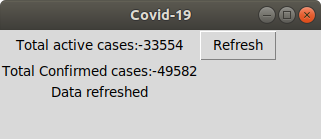GeeksforGeeks App
Open AppBrowser
Continue

# How to create a COVID19 Data Representation GUI?

Prerequisites: Python Requests, Python GUI – tkinter
Sometimes we just want a quick fast tool to really tell whats the current update, we just need a bare minimum of data. Web scraping deals with taking some data from the web and then processing it and displaying the relevant content in a short and crisp manner.
What the code is doing ?

• First we are using Tkinter Library to make GUI required for our script
• We are using requests Library to get the data from the unofficial api
• Then we are displaying the data we need in this case its Total active cases: and confirmed cases

below is the implementation.

## Python3

 `import` `requests``import` `json``from` `tkinter ``import` `*` `window ``=` `Tk()` `# creating the Box``window.title(``"Covid-19"``)` `# Determining the size of the Box``window.geometry(``'220x70'``)` `# Including labels``lbl ``=` `Label(window,``            ``text ``=``"Total active cases:-......"``)``lbl1 ``=` `Label(window,``             ``text ``=``"Total confirmed cases:-..."``)` `lbl.grid(column ``=` `1``, row ``=` `0``)``lbl1.grid(column ``=` `1``, row ``=` `1``)``lbl2 ``=` `Label(window, text ``=``"")``lbl2.grid(column ``=` `1``, row ``=` `3``)`  `def` `clicked():``    ``# Opening the url and loading the``    ``# json data using json Library``    ``url ``=` `"https://api.covid19india.org / data.json"``    ``page ``=` `requests.get(url)``    ``data ``=` `json.loads(page.text)``    ` `    ``lbl.configure(text ``=``"Total active cases:-"``                  ``+` `data[``"statewise"``][``0``][``"active"``])``    ` `    ``lbl1.configure(text ``=``"Total Confirmed cases:-"``                   ``+` `data[``"statewise"``][``0``][``"confirmed"``])``    ` `    ``lbl2.configure(text ``=``"Data refreshed"``)` `btn ``=` `Button(window, text ``=``"Refresh"``, command ``=` `clicked)``btn.grid(column ``=` `2``, row ``=` `0``)` `window.mainloop()`

Output:My Personal Notes arrow_drop_up